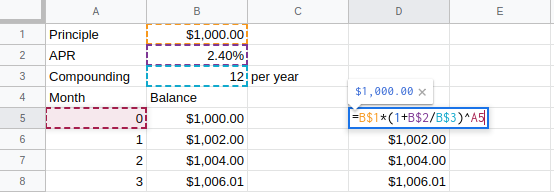## MTH098 Quantitative Literacy 2Math for "Real" Life

### Rational Equations

Solve for x in 3/(x+2)-4/(x-5)=2/(x^2-3x-10)

Factor all denominators:
3/(x+2)-4/(x-5)=2/((x+2)(x-5))

Find the lowest common denominator:
LCD=(x+2)(x-5)

Multiply all terms in the equation by the LCD:
(x+2)(x-5)*3/(x+2)-(x+2)(x-5)*4/(x-5) =(x+2)(x-5)*2/((x+2)(x-5))

Divide out the common factors:
cancel((x+2))(x-5)*3/cancel(x+2)-(x+2)cancel((x-5))*4/cancel(x-5) =cancel((x+2)(x-5))*2/cancel((x+2)(x-5))

The equation simplifies to:
3(x-5)-4(x+2)=2

Simplify:
3x-15-4x-8=2
x=-25

CHECK:
3/((-25)+2)-4/((-25)-5)=-3/23+2/15=(-45+46)/(23*15)=1/345
2/((-25)^2-3(-25)-10)=2/(625+75-10)=1/690=1/345
It checks!!!

### Balancing Blood Alcohol

Problem Situation: Calculating Blood Alcohol Content

BAC = -0.015*"hours"+(2.84*"drinks")/("Weight"*"gender")

Hours Blood Alcohol Content
0 0.116
1 0.101
2 0.086
3 0.071
4 0.056
5 0.041Solve for x in sqrt(10-x^2)-x=2

sqrt(10-x^2)=x+2

Square both sides:
(sqrt(10-x^2))^2=(x+2^2)

Clean up:
10-x^2=(x+2)(x+2)
10-x^2=x^2+4x+4

Collect all terms on one side:
0=2x^2+4x-6

Pray you can factor, or use Quadratic Formula:
0=2(x^2+2x-3)
0=2(x+3)(x-1)
So, x=-3 or x=1

CHECK:
sqrt(10-(-3)^2)-(-3)=sqrt(1)+3=4, but NOT 2
x=-3 does not check!?!
sqrt(10-(1)^2)-(1)=sqrt(9)-1=3-1=2, This Checks!!!
So, only x=1 is a solution

### Compunding Interest Makes Cents

Problem Situation: The Value of a CD

Suppose you invest \$1,000 principal in a two-year CD, advertised with an annual percentage rate (APR) of 2.4%, where compounding occurs monthly.

I=P*r*t##### Disclaimer:

You should check with your advisor before taking a math class leading to calculus. Algebra classes are known to cause stress, anger, depression, and occasionally rashes, as well as lead to calculus. Please consult a physician if negative symptoms persist.

Sample Course

Sample Course